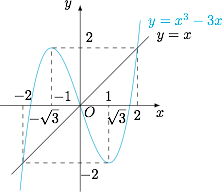# 每日一题分段函数的零点

$(1)$ 若 $f(x)$ 有两个零点，则实数 $a$ 的取值范围是_______；

$(2)$ 若 $a\leqslant -2$，则满足 $f(x)+f(x-1)>-3$ 的 $x$ 的取值范围是_______．$(2)$ 当 $a\leqslant -2$ 时，函数 $f(x)$ 在 $\mathbb{R}$ 上单调递增，令$g(x)=f(x)+f(x-1),$则 $g(x)$ 在 $\mathbb{R}$ 上单调递增．由于 $g(-1)=-3$，故 $x>-1$．